# Statistics review 5: Comparison of means

## Abstract

The present review introduces the commonly used t-test, used to compare a single mean with a hypothesized value, two means arising from paired data, or two means arising from unpaired data. The assumptions underlying these tests are also discussed.

## Introduction

Previous reviews in this series have introduced the principals behind the calculation of confidence intervals and hypothesis testing. The present review covers the specific case of comparing means in rather more detail. Comparison of means arises in many different formats, and there are various methods available for dealing with each of these. Some of the simpler cases are covered in this review, namely comparison of a single observed mean with some hypothesized value, comparison of two means arising from paired data, and comparison of two means from unpaired data. All of these comparisons can be made using appropriate confidence intervals and t-tests as long as certain assumptions are met (see below). Future reviews will introduce techniques that can be used when the assumptions of the t-test are not valid or when the comparison is between three or more groups.

Of the three cases covered in this review, comparison of means from unpaired data is probably the most common. However, the single mean and paired data cases are introduced first because the t-test in these cases is more straightforward.

## Comparison of a single mean with a hypothesized value

This situation is not very common in practice but on occasion it may be desirable to compare a mean value from a sample with some hypothesized value, perhaps from external standards. As an example, consider the data shown in Table 1. These are the haemoglobin concentrations of 15 UK adult males admitted into an intensive care unit (ICU). The population mean haemoglobin concentration in UK males is 15.0 g/dl. Is there any evidence that critical illness is associated with an acute anaemia?

The mean haemoglobin concentration of these men is 9.7 g/dl, which is lower than the population mean. However, in practice any sample of 15 men would be unlikely to have a mean haemoglobin of exactly 15.0 g/dl, so the question is whether this difference is likely to be a chance finding, due to random variation, or whether it is the result of some systematic difference between the men in the sample and those in the general population. The best way to determine which explanation is most likely is to calculate a confidence interval for the mean and to perform a hypothesis test.

The standard deviation (SD) of these data is 2.2 g/dl, and so a 95% confidence interval for the mean can be calculated using the standard error (SE) in the usual way. The SE in this case is 2.2/√15 = 0.56 and the corresponding 95% confidence interval is as follows.

9.7 ± 2.14 × 0.56 = 9.7 ± 1.19 = (8.5, 10.9)

Note that the multiplier, in this case 2.14, comes from the tdistribution because the sample size is small (for a fuller explanation of this calculation, see Statistics review 2 from this series). This confidence interval gives the range of likely values for the mean haemoglobin concentration in the population from which these men were drawn. In other words, assuming that this sample is representative, it is likely that the true mean haemoglobin in the population of adult male patients admitted to ICUs is between 8.5 and 10.9 g/dl. The haemoglobin concentration in the general population of adult men in the UK is well outside this range, and so the evidence suggests that men admitted to ICUs may genuinely have haemoglobin concentrations that are lower than the national average.

Exploration of how likely it is that this difference is due to chance requires a hypothesis test, in this case the one sample t-test. The t-test formally examines how far the estimated mean haemoglobin of men admitted to ICU, in this case 9.7 g/dl, lies from the hypothesized value of 15.0 g/dl. The null hypothesis is that the mean haemoglobin concentration of men admitted to ICU is the same as the standard for the adult male UK population, and so the further away the sample mean is from this hypothesized value, the less likely it is that the difference arose by chance.

The t statistic, from which a P value is derived, is as follows.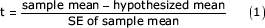In other words, t is the number of SEs that separate the sample mean from the hypothesized value. The associated P value is obtained by comparison with the t distribution introduced in Statistics review 2, with larger t statistics (regardless of sign) corresponding to smaller P values. As previously described, the shape of the t distribution is determined by the degrees of freedom, which, in the case of the one sample t-test, is equal to the sample size minus 1.

The t statistic for the haemoglobin example is as follows.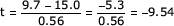In other words, the observed mean haemoglobin concentration is 9.54 SEs below the hypothesized mean. Tabulated values indicate how likely this is to occur in practice, and for a sample size of 15 (corresponding to 14 degrees of freedom) the P value is less than 0.0001. In other words, it is extremely unlikely that the mean haemoglobin in this sample would differ from that in the general population to this extent by chance alone. This may indicate that there is a genuine difference in haemoglobin concentrations in men admitted to the ICU, but as always it is vital that this result be interpreted in context. For example, it is important to know how this sample of men was selected and whether they are representative of all UK men admitted to ICUs.

Note that the P value gives no indication of the size of any difference; it merely indicates the probability that the difference arose by chance. In order to assess the magnitude of any difference, it is essential also to have the confidence interval calculated above.

## Comparison of two means arising from paired data

A special case of the one sample t-test arises when paired data are used. Paired data arise in a number of different situations, such as in a matched case–control study in which individual cases and controls are matched to each other, or in a repeat measures study in which some measurement is made on the same set of individuals on more than one occasion (generally under different circumstances). For example, Table 2 shows central venous oxygen saturation in 10 patients on admission and 6 hours after admission to an ICU.

The mean admission central venous oxygen saturation was 52.4% as compared with a mean of 59.2% after 6 hours, corresponding to an increase of 6.8%. Again, the question is whether this difference is likely to reflect a genuine effect of admission and treatment or whether it is simply due to chance. In other words, the null hypothesis is that the mean central venous oxygen saturation on admission is the same as the mean saturation after 6 hours. However, because the data are paired, the two sets of observations are not independent of each other, and it is important to account for this pairing in the analysis. The way to do this is to concentrate on the differences between the pairs of measurements rather than on the measurements themselves.

The differences between the admission and post-admission central venous oxygen saturations are given in the rightmost column of Table 2, and the mean of these differences is 6.8%. In these terms, the null hypothesis is that the mean of the differences in central venous oxygen saturation is zero. The appropriate t-test therefore compares the observed mean of the differences with a hypothesized value of 0. In other words, the paired t-test is simply a special case of the single sample t-test described above.

The t statistic for the paired t-test is as follows.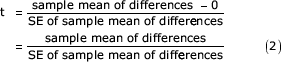The SD of the differences in the current example is 7.5, and this corresponds to a SE of 7.5/√10 = 2.4. The t statistic is therefore t = 6.8/2.4 = 2.87, and this corresponds to a P value of 0.02 (based on a t distribution with 10-1 = 9 degrees of freedom). In other words, there is some evidence to suggest that admission to ICU and subsequent treatment may increase central venous oxygen saturation beyond the level expected by chance.

However, the P value in isolation gives no information about the likely size of any effect. As indicated above, this is rectified by calculating a 95% confidence interval from the mean and SE of the differences. In this case the 95% confidence interval is as follows.

6.8 ± 2.26 × 2.4 = 6.8 ± 5.34 = (1.4, 12.2)

This indicates that the true increase in central venous oxygen saturation due to ICU admission and treatment in the population is probably between 1.4% and 12.2%. The decision as to whether this difference is likely to be important in practice should be based on the statistical evidence in combination with other relevant clinical factors. However, it is worth noting that the confidence interval excludes 0 (the expected difference if the null hypothesis were true); thus, although the increase may be small (1.4%), it is unlikely that the effect is to decrease saturation.

## Comparison of two means arising from unpaired data

The most common comparison is probably that of two means arising from unpaired data (i.e. comparison of data from two independent groups). For example, consider the results from a recently published trial that compared early goal-directed therapy with standard therapy in the treatment of severe sepsis and septic shock . A total of 263 patients were randomized and 236 completed 6 hours of treatment. The mean arterial pressures after 6 hours of treatment in the standard and early goal-directed therapy groups are shown in Table 3.

Note that the authors of this study also collected information on baseline mean arterial pressure and examined the 6-hour pressures in the context of these (using a method known as analysis of covariance) . In practice this is a more appropriate analysis, but for illustrative purposes the focus here is on 6-hour mean arterial pressures only.

It appears that the mean arterial pressure was 14 mmHg higher in the early goal-directed therapy group. The 95% confidence intervals for the mean arterial pressure in the two groups are as follows.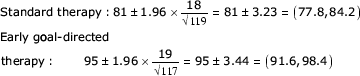There is no overlap between the two confidence intervals and, because these are the ranges in which the true population values are likely to lie, this supports the notion that there may be a difference between the two groups. However, it is more useful to estimate the size of any difference directly, and this can be done in the usual way. The only difference is in the calculation of the SE.

In the paired case attention is focused on the mean of the differences; in the unpaired case interest is in the difference of the means. Because the sample sizes in the unpaired case may be (and indeed usually are) different, the combined SE takes this into account and gives more weight to the larger sample size because this is likely to be more reliable. The pooled SD for the difference in means is calculated as follows: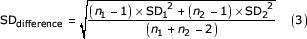where SD1 and SD2 are the SDs in the two groups and n1 and n2 are the two sample sizes. The pooled SE for the difference in means is then as follows.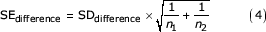This SE for the difference in means can now be used to calculate a confidence interval for the difference in means and to perform an unpaired t-test, as above.

The pooled SD in the early goal-directed therapy trial example is: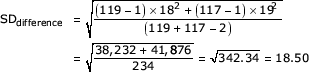and the corresponding pooled SE is: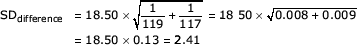The difference in mean arterial pressure between the early goal-directed and standard therapy groups is 14 mmHg, with a corresponding 95% confidence interval of 14 ± 1.96 × 2.41= (9.3, 18.7) mmHg. If there were no difference in the mean arterial pressures of patients randomized to early goal-directed and standard therapy then the difference in means would be close to 0. However, the confidence interval excludes this value and suggests that the true difference is likely to be between 9.3 and 18.7 mmHg.

To explore the likely role of chance in explaining this difference, an unpaired t-test can be performed. The null hypothesis in this case is that the means in the two populations are the same or, in other words, that the difference in the means is 0. As for the previous two cases, a t statistic is calculated.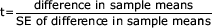A P value may be obtained by comparison with the t distribution on n1 + n2 - 2 degrees of freedom. Again, the larger the t statistic, the smaller the P value will be.

In the early goal-directed therapy example t = 14/2.41 = 5.81, with a corresponding P value less than 0.0001. In other words, it is extremely unlikely that a difference in mean arterial pressure of this magnitude would be observed just by chance. This supports the notion that there may be a genuine difference between the two groups and, assuming that the randomization and conduct of the trial was appropriate, this suggests that early goal-directed therapy may be successful in raising mean arterial pressure by between 9.3 and 18.7 mmHg. As always, it is important to interpret this finding in the context of the study population and, in particular, to consider how readily the results may be generalized to the general population of patients with severe sepsis or septic shock.

## Assumptions and limitations

In common with other statistical tests, the t-tests presented here require that certain assumptions be made regarding the format of the data. The one sample t-test requires that the data have an approximately Normal distribution, whereas the paired t-test requires that the distribution of the differences are approximately Normal. The unpaired t-test relies on the assumption that the data from the two samples are both Normally distributed, and has the additional requirement that the SDs from the two samples are approximately equal.

Formal statistical tests exist to examine whether a set of data are Normal or whether two SDs (or, equivalently, two variances) are equal , although results from these should always be interpreted in the context of the sample size and associated statistical power in the usual way. However, the t-test is known to be robust to modest departures from these assumptions, and so a more informal investigation of the data may often be sufficient in practice.

If assumptions of Normality are violated, then appropriate transformation of the data (as outlined in Statistics review 1) may be used before performing any calculations. Similarly, transformations may also be useful if the SDs are very different in the unpaired case . However, it may not always be possible to get around these limitations; where this is the case, there are a series of alternative tests that can be used. Known as nonparametric tests, they require very few or very limited assumptions to be made about the format of the data, and can therefore be used in situations where classical methods, such as t-tests, may be inappropriate. These methods will be the subject of the next review, along with a discussion of the relative merits of parametric and nonpara-metric approaches.

Finally, the methods presented here are restricted to the case where comparison is to be made between one or two groups. This is probably the most common situation in practice but it is by no means uncommon to want to explore differences in means across three or more groups, for example lung function in nonsmokers, current smokers and ex-smokers. This requires an alternative approach that is known as analysis of variance (ANOVA), and will be the subject of a future review.

## Abbreviations

ICU:

intensive care unit

SD:

standard deviation

SE:

standard error.

## References

1. Rivers E, Nguyen B, Havstad S, Ressler J, Muzzin A, Knoblich B, Peterson E, Tomlanovich M: Early goal-directed therapy in the treatment of severe sepsis and septic shock. N Engl J Med 2001, 345: 1368-1377. 10.1056/NEJMoa010307

2. Altman DG: Practical Statistics for Medical Research. London, UK: Chapman & Hall, 1991.

3. Kirkwood BR: Essentials of Medical Statistics. Oxford, UK: Blackwell Science Ltd, 1988.

Authors

None declared.

## Rights and permissions

Reprints and Permissions

Whitley, E., Ball, J. Statistics review 5: Comparison of means. Crit Care 6, 424 (2002). https://doi.org/10.1186/cc1548